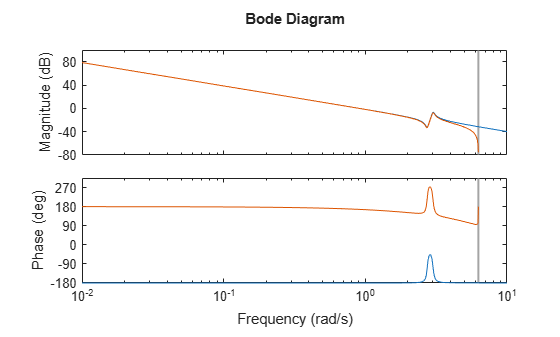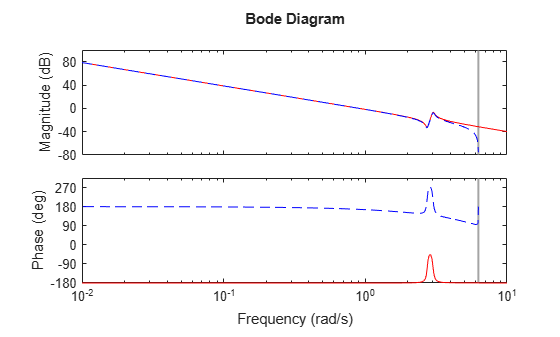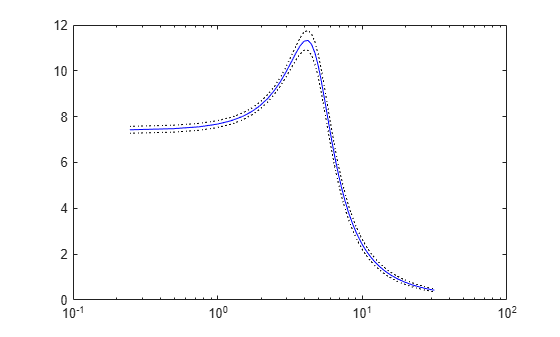Documentation

bode

Bode plot of frequency response, or magnitude and phase data

Description

example

bode(sys) creates a Bode plot of the frequency response of a dynamic system model sys. The plot displays the magnitude (in dB) and phase (in degrees) of the system response as a function of frequency. bode automatically determines frequencies to plot based on system dynamics.

If sys is a multi-input, multi-output (MIMO) model, then bode produces an array of Bode plots, each plot showing the frequency response of one I/O pair.

example

bode(sys1,sys2,...,sysN) plots the frequency response of multiple dynamic systems on the same plot. All systems must have the same number of inputs and outputs.

example

bode(sys1,LineSpec1,...,sysN,LineSpecN) specifies a color, linestyle, and marker for each system in the plot.

example

bode(___,w) plots system responses for frequencies specified by w.

• If w is a cell array of the form {wmin,wmax}, then bode plots the response at frequencies ranging between wmin and wmax.

• If w is a vector of frequencies, then bode plots the response at each specified frequency.

You can use w with any of the input-argument combinations in previous syntaxes.

example

[mag,phase,wout] = bode(sys) returns the magnitude and phase of the response at each frequency in the vector wout. The function automatically determines frequencies in wout based on system dynamics. This syntax does not draw a plot.

example

[mag,phase,wout] = bode(sys,w) returns the response data at the frequencies specified by w.

• If w is a cell array of the form {wmin,wmax}, then wout contains frequencies ranging between wmin and wmax.

• If w is a vector of frequencies, then wout = w.

example

[mag,phase,wout,sdmag,sdphase] = bode(sys,w) also returns the estimated standard deviation of the magnitude and phase values for the identified model sys. If you omit w, then the function automatically determines frequencies in wout based on system dynamics.

Examples

collapse all

Create a Bode plot of the following continuous-time SISO dynamic system.

$H\left(s\right)=\frac{{s}^{2}+0.1s+7.5}{{s}^{4}+0.12{s}^{3}+9{s}^{2}}.$

H = tf([1 0.1 7.5],[1 0.12 9 0 0]);
bode(H)bode automatically selects the plot range based on the system dynamics.

Create a Bode plot over a specified frequency range. Use this approach when you want to focus on the dynamics in a particular range of frequencies.

H = tf([-0.1,-2.4,-181,-1950],[1,3.3,990,2600]);
bode(H,{1,100})
grid onThe cell array {1,100} specifies the minimum and maximum frequency values in the Bode plot. When you provide frequency bounds in this way, the function selects intermediate points for frequency response data.

Alternatively, specify a vector of frequency points to use for evaluating and plotting the frequency response.

w = [1 5 10 15 20 23 31 40 44 50 85 100];
bode(H,w,'.-')
grid onbode plots the frequency response at the specified frequencies only.

Compare the frequency response of a continuous-time system to an equivalent discretized system on the same Bode plot.

Create continuous-time and discrete-time dynamic systems.

H = tf([1 0.1 7.5],[1 0.12 9 0 0]);
Hd = c2d(H,0.5,'zoh');

Create a Bode plot that displays both systems.

bode(H,Hd)The Bode plot of a discrete-time system includes a vertical line marking the Nyquist frequency of the system.

Specify the line style, color, or marker for each system in a Bode plot using the LineSpec input argument.

H = tf([1 0.1 7.5],[1 0.12 9 0 0]);
Hd = c2d(H,0.5,'zoh');
bode(H,'r',Hd,'b--')The first LineSpec, 'r', specifies a solid red line for the response of H. The second LineSpec, 'b--', specifies a dashed blue line for the response of Hd.

Compute the magnitude and phase of the frequency response of a SISO system.

If you do not specify frequencies, bode chooses frequencies based on the system dynamics and returns them in the third output argument.

H = tf([1 0.1 7.5],[1 0.12 9 0 0]);
[mag,phase,wout] = bode(H);

Because H is a SISO model, the first two dimensions of mag and phase are both 1. The third dimension is the number of frequencies in wout.

size(mag)
ans = 1×3

1     1    42

length(wout)
ans = 42

Thus, each entry along the third dimension of mag gives the magnitude of the response at the corresponding frequency in wout.

For this example, create a 2-output, 3-input system.

rng(0,'twister'); % For reproducibility

For this system, bode plots the frequency responses of each I/O channel in a separate plot in a single figure.

bode(H)Compute the magnitude and phase of these responses at 20 frequencies between 1 and 10 radians.

w = logspace(0,1,20);
[mag,phase] = bode(H,w);

mag and phase are three-dimensional arrays, in which the first two dimensions correspond to the output and input dimensions of H, and the third dimension is the number of frequencies. For instance, examine the dimensions of mag.

size(mag)
ans = 1×3

2     3    20

Thus, for example, mag(1,3,10) is the magnitude of the response from the third input to the first output, computed at the 10th frequency in w. Similarly, phase(1,3,10) contains the phase of the same response.

Compare the frequency response of a parametric model, identified from input/output data, to a nonparametric model identified using the same data.

Identify parametric and nonparametric models based on data.

w = linspace(0,10*pi,128);
sys_np = spa(z2,[],w);
sys_p = tfest(z2,2);

Using the spa and tfest commands requires System Identification Toolbox™ software.

sys_np is a nonparametric identified model. sys_p is a parametric identified model.

Create a Bode plot that includes both systems.

bode(sys_np,sys_p,w);
legend('sys-np','sys-p')You can display the confidence region on the Bode plot by right-clicking the plot and selecting Characteristics > Confidence Region.

Compute the standard deviation of the magnitude and phase of an identified model. Use this data to create a 3σ plot of the response uncertainty.

Identify a transfer function model based on data. Obtain the standard deviation data for the magnitude and phase of the frequency response.

sys_p = tfest(z2,2);
w = linspace(0,10*pi,128);
[mag,ph,w,sdmag,sdphase] = bode(sys_p,w);

Using the tfest command requires System Identification Toolbox™ software.

sys_p is an identified transfer function model. sdmag and sdphase contain the standard deviation data for the magnitude and phase of the frequency response, respectively.

Use the standard deviation data to create a 3σ plot corresponding to the confidence region.

mag = squeeze(mag);
sdmag = squeeze(sdmag);
semilogx(w,mag,'b',w,mag+3*sdmag,'k:',w,mag-3*sdmag,'k:');Input Arguments

collapse all

Dynamic system, specified as a SISO or MIMO dynamic system model or array of dynamic system models. Dynamic systems that you can use include:

• Continuous-time or discrete-time numeric LTI models, such as tf, zpk, or ss models.

• Generalized or uncertain LTI models such as genss or uss models. (Using uncertain models requires Robust Control Toolbox™ software.)

• For tunable control design blocks, the function evaluates the model at its current value for both plotting and returning frequency response data.

• For uncertain control design blocks, the function plots the nominal value and random samples of the model. When you use output arguments, the function returns frequency response data for the nominal model only.

• Frequency-response data models such as frd models. For such models, the function plots the response at frequencies defined in the model.

• Identified LTI models, such as idtf, idss, or idproc models. For such models, the function can also plot confidence intervals and return standard deviations of the frequency response. See Bode Plot of Identified Model. (Using identified models requires System Identification Toolbox™ software.)

If sys is an array of models, the function plots the frequency responses of all models in the array on the same axes.

Line style, marker, and color, specified as a string or vector of one, two, or three characters. The characters can appear in any order. You do not need to specify all three characteristics (line style, marker, and color). For example, if you omit the line style and specify the marker, then the plot shows only the marker and no line. For more information about configuring this argument, see the LineSpec input argument of the plot function.

Example: 'r--' specifies a red dashed line

Example: '*b' specifies blue asterisk markers

Example: 'y' specifies a yellow line

Frequencies at which to compute and plot frequency response, specified as the cell array {wmin,wmax} or as a vector of frequency values.

• If w is a cell array of the form {wmin,wmax}, then the function computes the index at frequencies ranging between wmin and wmax.

• If w is a vector of frequencies, then the function computes the index at each specified frequency. For example, use logspace to generate a row vector with logarithmically spaced frequency values.

Specify frequencies in units of rad/TimeUnit, where TimeUnit is the TimeUnit property of the model.

Output Arguments

collapse all

Magnitude of the system response in absolute units, returned as a 3-D array. The dimensions of this array are (number of system outputs) × (number of system inputs) × (number of frequency points).

To convert the magnitude from absolute units to decibels, use:

magdb = 20*log10(mag)

Phase of the system response in degrees, returned as a 3-D array. The dimensions of this array are (number of outputs) × (number of inputs) × (number of frequency points).

Frequencies at which the function returns the system response, returned as a column vector. The function chooses the frequency values based on the model dynamics, unless you specify frequencies using the input argument w.

Frequency values are in radians per TimeUnit, where TimeUnit is the value of the TimeUnit property of sys.

Estimated standard deviation of the magnitude of the response at each frequency point, returned as a 3-D array. sdmag has the same dimensions as mag.

If sys is not an identified LTI model, sdmag is [].

Estimated standard deviation of the phase of the response at each frequency point, returned as a 3-D array. sdphase has the same dimensions as phase.

If sys is not an identified LTI model, sdphase is [].

Tips

• When you need additional plot customization options, use bodeplot instead.

Algorithms

bode computes the frequency response as follows:

1. Compute the zero-pole-gain (zpk) representation of the dynamic system.

2. Evaluate the gain and phase of the frequency response based on the zero, pole, and gain data for each input/output channel of the system.

• For continuous-time systems, bode evaluates the frequency response on the imaginary axis s = and considers only positive frequencies.

• For discrete-time systems, bode evaluates the frequency response on the unit circle. To facilitate interpretation, the command parameterizes the upper half of the unit circle as:

$z={e}^{j\omega {T}_{s}},\text{ }0\le \omega \le {\omega }_{N}=\frac{\pi }{{T}_{s}},$

where Ts is the sample time and ωN is the Nyquist frequency. The equivalent continuous-time frequency ω is then used as the x-axis variable. Because $H\left({e}^{j\omega {T}_{s}}\right)$ is periodic with period 2ωN, bode plots the response only up to the Nyquist frequency ωN. If sys is a discrete-time model with unspecified sample time, bode uses Ts = 1.